# Geometry

#### Coordinate Geometry - 2

Triangles - Mid Point Theorem and Similarity 1

Mid Point theorem and Similarity

Basic Proportionality Theorem/ Thales Theorem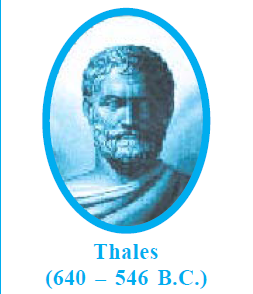This is known as the basic proportionality theorem or sometimes by Thales theorem. But what does it exactly mean?

Consider a triangle ABC. A line is drawn parallel to the side BC and this line intersects the side AB at point D and AC at point E. Then,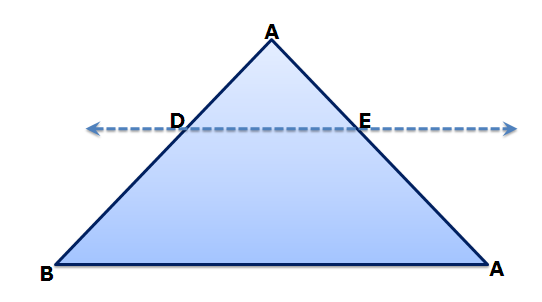Converse of Basic Proportionality Theorem:

It states that if a line divides any two sides of a triangle in the same ratio, then the line is parallel to the third side.

Q1. In the given figure DF || BG , FE|| GC, AD = 3, BD = 5, AE = 4.5. What is the length of EC?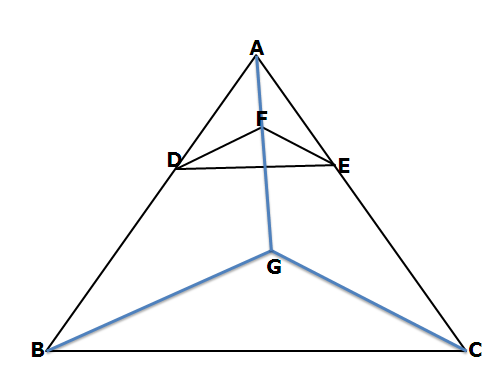1. 5          2. 6           3. 7.5         4. 9

Solution:

Consider ΔABG, DF || BG,

So,  AD/BD = AF/FG   (1) { Basic Proportionality Theorem}

Consider ΔAGC, FE || GC,

So, AF/FG = AE/EC     (2) { Basic Proportionality Theorem}

From (1) and (2), we get

Therefore   3/5 = 4.5/ EC   or EC = 7.5

Q2. In ΔABC, AD is a line that intersects BC at point D such that BD:DC is 3:1. Also BF is a line that intersects AD at point E and F lies on AC such that AE : ED is 2:3. If the length of AC is 15 units, then the value of AF is –

1. 2             2. 5             3. 6           4. 7.5

Solution:- First lets draw the figure.  Now we shall do a little construction. Draw a line DG parallel to EF.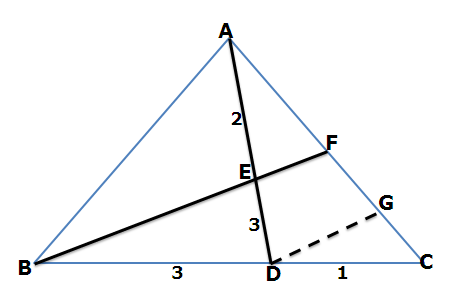Consider ΔCBF, GD is parallel to BF

So, CD/DB = CG/GF   { Basic Proportionality Theorem}

So,   CG = 1/3 FG

If CG = x, then FG = 3x

Now, consider ΔADG, EF is parallel to GD

So, AE/ED = AF/FG

2/3  = AF/3x    or AF = 2x

So, AC = AF + FG + GC = 2x + 3x + x = 6x

Thus, 6x = 15  or x = 2.5

Hence, AF = 2.5 * 2 = 5 units.

Example 3: In ?ABC, BE is the angle bisector of angle ABC. AD is drawn perpendicular to BE, intersecting it at the point D. From D a line is drawn parallel to BC intersecting AC at F. If the length of AC is 18 units, then what is the length of AF?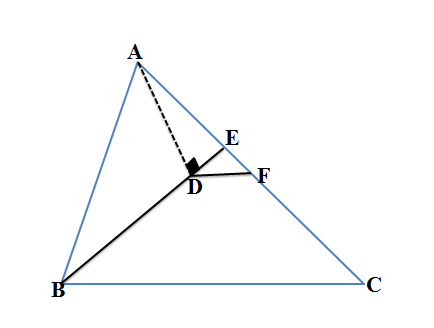Solution: Extend DF so that it intersects AB at G as shown in the figure.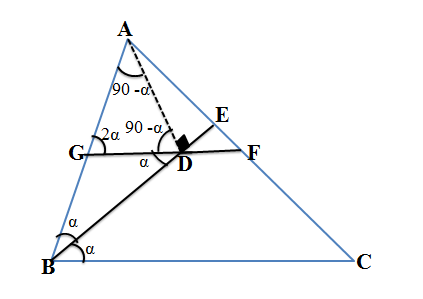As BE is the angle bisector and GD || BC, So angle CBD = angle DBC = angle GDB = α

Also angle AGD = 2α ( Exterior Angle in ?GBD)

Thus, BG = GD  (1) ( Sides opposite to equal angles)

Consider ?AGD,

Angle GDA = 90 –α { As AD is perpendicular to BE}

Angle AGD = 2α , this gives angle GAD = 90 –α

So GD = AG        (2)

From (1) and (2) we get

BG = AG i.e. AG/BG = 1, so AF/FC should also be equal to 1 ( From Thale’s Theorem)

Thus, AF = FC = 9 { As AC = 18 units}

Midpoint Theorem:

In a triangle ABC, if D and E are the midpoints of AB and AC respectively, then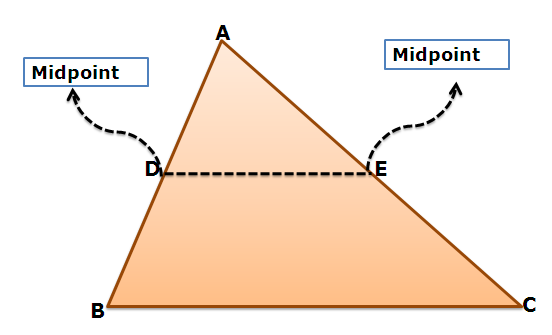Corollory:

If a line is drawn from a midpoint of one side and parallel to the base of a triangle, then it will pass through the midpoint of the other side.

Q3. D and E are the mid-points of AB and AC of ΔABC; BC is produced to any point P; DE, DP and EP are joined. Then,

1. ΔPED = ¼ ΔABC

2. ΔPED = ΔBEC

4. ΔBDE = ΔBEC

Solution: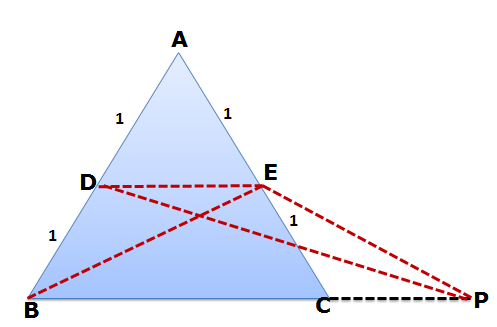As D and E are the midpoints, so DE =1/2 * BC and also DE|| BC.

Consider ΔAEB. As AD:BD is 1:1.

So area(ΔADE) =area(ΔBED) = a (Suppose)

Now lets consider ΔBED and ΔDEP, Here both the triangles are on the same base and between the same parallels. Hence the areas of both these triangles are equal.

Thus, area(ΔADE) = area(ΔPED) = a (1)

Also E is the midpoint of AC. Hence area(ΔABE) = area(ΔBEC) = 2a.

Hence area (ΔABC) = 2a+2a = 4a   (2)

From (1) and (2), we get

Area(ΔPED) = ¼ * Area(ΔABC)

Q4. XYZ is a triangle with side lengths XY =8,YZ =13 and XZ= 7 units. ΔX1Y1Z1 is a triangle formed by joining the midpoints of sides XY, YZ and ZX. Similarly ΔX2Y2Z2 is formed by joining the midpoints of sides X1Y1, Y2Z2 and X2Z2. This process is continued infinitely. What is the sum of the perimeters of all these triangles so formed?

Solution: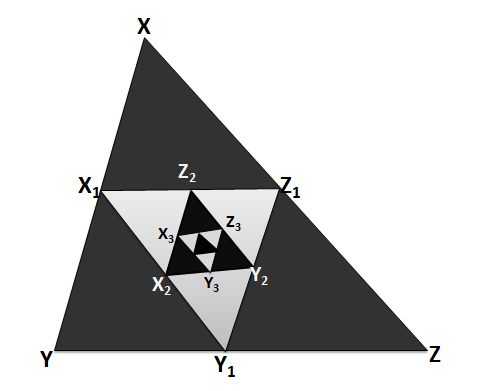As X1 and Y1 are the midpoints of XY and YZ, so by midpoint theorem X1Y1 = ½ XZ = 7/2 units

Similarly Y1 and Z1 are midpoints of YZ and XZ, so by midpoint theorem Y1Z1 = ½ XY = 4

Similarly X1 and Z1 are midpoints of XYand XZ, so by midpoint theorem X1Z1 = ½ YZ = 13/2

Also Per(ΔXYZ) = 7+8+13 = 28

Thus, Per(ΔX1Y1Z1) = 7/2  + 4 + 13/2  = 28/2 = 14

Similarly Per(ΔX2Y2Z2) = 14/2 = 7

Per (ΔX3Y3Z3) = 7/2

Sum of all these perimeters = 28 + 14+ 7 + 7/2 + ……………………….Similarity:

In the study of triangles, the idea of similarity constitutes a very important and a fundamental concept. Various problems involve application of similarity. But what exactly is similarity and what is the difference between similarity and congruency?

When we say two figures are congruent then we mean that both the figures are exactly the same in size and in shape. Or in other words two congruent figures are identical or can be superimposed on each other.

On the other hand two figures are similar when they have the same shape but not necessarily the same size. eg. Any two circles are always similar as they have the same shape. If their radius is also the same, then we will call them congruent circles.We shall confine our study to the similarity of triangles.

If two triangles are similar to each other, then their corresponding angles are equal and their corresponding sides are proportional to each other.

Consider two triangles ABC and DEF. If they are similar to each other, then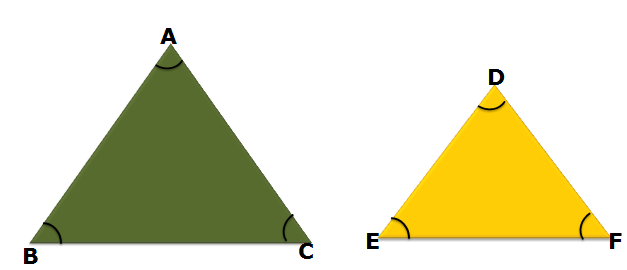1. Corresponding angles are equal, i.e. A = D, B = E  and C = F

2. Corresponding sides are in the same ratio

i.e.  AB/DE=BC/EF=AC/DF

Let this ratio of sides be k. Then the perimeters of both the triangles are also in the ratio of ‘k’. Also the corresponding medians, altitudes and angle bisectors, inradius and circumradius are in the ratio of ‘k’.

On the other hand the ratio of areas of these triangles is k2. i.e. the areas are in the ratio of square of the ratio of corresponding sides.

Applying similarity can be quite confusing at times and one might take wrong ratio of sides. So first consider those angles which are equal and then take the ratio of sides opposite to these angles.

Here A = D. Side opposite to A is BC and the side opposite to D is EF. Thus, we have taken the ratio of BC to EF {Corresponding sides}. Similarly for the other sides.

Q5. ΔABC and ΔDEF are similar and their areas be respectively 64 cm2 and 121 cm2. If EF = 15.4 cm, BC is

(A) 12.3 cm

(B)11.2 cm

(C) 12.1 cm

(D) 11 cm

Solution:  ΔABC and ΔDEF are similar to each other. Let the ratio of their sides is ‘k’ i.e. AB/DE = BC/EF = AC/DF = k

So, area of ΔABC/area of ΔDEF = 64/121  = k2

Or k = 8/11

Thus, BC/EF = 8/11   or BC = (8/11)*15.4 = 11.2

Option (B) is correct

Q6. The perimeters of two similar triangles ΔABC and ΔPQR are 36 cm and 24 cm respectively. If PQ = 10cm, then AB is :

(A) 25 cm

(B) 10 cm

(C) 15 cm

(D) 20 cm

Solution : As the triangles are similar, the ratio of their sides is the same as the ratio of their perimeters.

Thus,  AB/PQ = Per(ΔABC)/Per(ΔPQR)=36/24 = 3/2

So, AB = 10*(3/2) = 15 cm

Option (C) is correct.

Q7. In the given figure DE || FG || BC. Also, DE:FG = 2:3 and AF:FB = 3:1.If area of ΔADE = 80, then the area of ΔEFC is-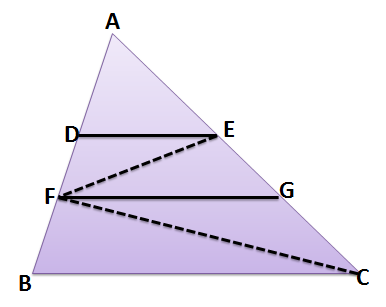Solution: Here DE || FG || BC. So ΔADF ~ ΔAFG ABC. { Why???? Because In parallel lines corresponding angles are equal and so all the corresponding angles of these three triangles are equal}

Consider ΔADF and ΔAFG, here DE:FG = 2:3 and so AD: AF is also 2:3 which means AD:DF = 2:1

So Area(DEF) =  40

{ Areas is divided in the same ratio as the base is divided}

So Area ΔAFG = 180   or Area(DEGF) = 180 -80 =100

Area (ΔGEF) = 100 – 40 = 60

The situation is as shown below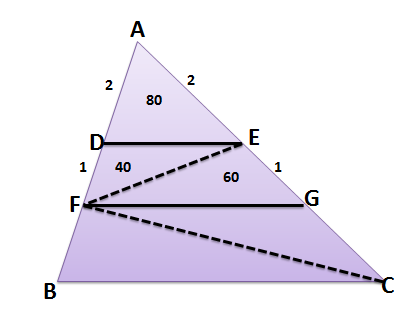Also AF:FB = 3:1 which means AG : GC = 3:1 { Basic Proportionality Theorem}

Thus EG: GC = 1:1

which means area(ΔCGF) = area(ΔEFG) = 60

Thus, area(ΔEFC) = 60+60 = 120 units.

Q8.  In the figure given below, ABC is a right triangle right angled at B and BDEF is a rectangle. If the inradius of ?ADE is 2 units while the inradius of ?CEF is 6 units and the perimeter of ?ADE is 12 units, then the area of rectangle BDEF is ?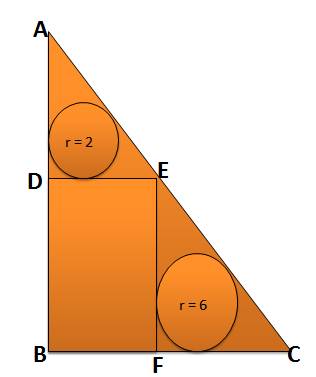Solution: BDEF is a rectangle , so DE || BC and so ?ADE ~ ?EFC ~ ?ABC

Area of ?ADE = 2*(12/2) = 12  { As semiperimeter = ½ * Perimeter}

Ratio of sides = Ratio of inradii { for similar triangles }

AD/EF = DE/FC = AE/EC = r1/r2 = 2/6  or 1/3

Thus, the lengths of sides of ?EFC are thrice the lengths of sides of ?ADE

Thus, ratio of areas of ?ADE and EFC is (1/3)2 i.e 1:9

Area of ?EFC = 9*12 = 108 units.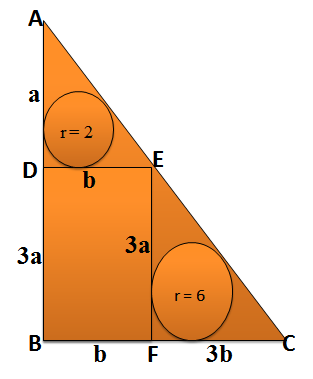Area of BDEF = Area(?ABC) – Area(?ADE) –Area(EFC)

= 192 – 12 – 108 = 72

9.  As shown in the figure, PQRS is a square kept on side BC of the triangle. If BC = 10 units and the area of ?ABC is 75 units, then what is the perimeter of PQRS?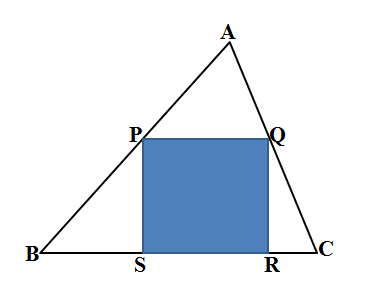Solution: As BC = 10 units and the area of ?ABC = 75, so height of the triangle is 15 units

Consider ?APQ and ?ABC. Both are similar to each other as PQ || BC

So, the sides of these two triangles will be in the same ratio as their heights.

Let the side length of the square be x units.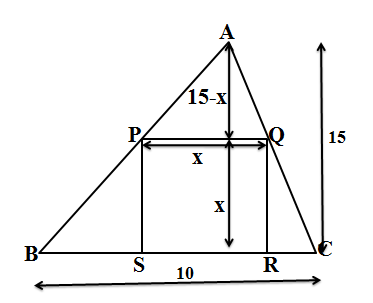So,   x/10 = (15-x)/15

Or 15x = 150 – 10x

Thus, x = 6 units

So, the perimeter of PQRS = 24 units

10.    In the figure shown, DF || BC.  If BC = 2EF and EF = 2DE, then what is the ratio of area of ?DEG to area of ? AEF?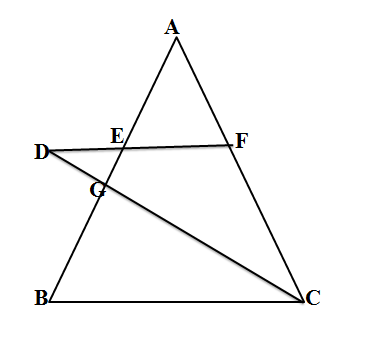Solution: Let DE = x, then EF = 2x and BC = 4x.

As DE || BC. So, ?DEG is similar to ?BGC

As DE: BC = 1:4, so their areas are in the ratio of 1:16.

Join GF.As DE : EF = 1:2 , so Area of ?GEF = 2a

Also  As DGE is similar to BGC, so DG : GC = 1:4

And so Area of ?DGF : ?GFC = 1:4   or Area of ?GFC = 12a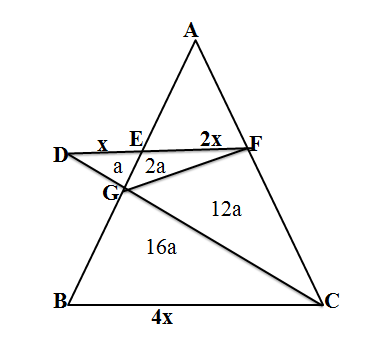As EF || BC, So ?AEF is similar to ?ABC.

EF : BC = 1:2 so their areas are in the ratio of 1:4.

As Area of EFCB = 2a+12a+16a = 30a, so area of ?AEF = 10a